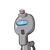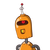# Show that any positive odd integer is of the form 6q+1, or 6q + 3, or 6q+5, where q is some integer.​

Show that any positive odd integer is of the form 6q+1, or 6q + 3, or 6q+5, where q is some integer.

### 2 thoughts on “Show that any positive odd integer is of the form 6q+1, or 6q + 3, or 6q+5, where q is some integer.<br />​”

1.Let a be a given integer.

On dividing a by 6 , we get q as the quotient and r as the remainder such that

a = 6q + r, r = 0,1,2,3,4,5

when r=0

a = 6q,even no

when r=1

a = 6q + 1, odd no

when r=2

a = 6q + 2, even no

when r = 3

a=6q + 3,odd no

when r=4

a=6q + 4,even no

when r=5,

a= 6q + 5 , odd no

Any positive odd integer is of the form 6q+1,6q+3 or 6q+5.

2.$$\huge\mathcal\colorbox{pink}{{\color{b}{✿Yøur-AñsWēR♡}}}$$

According to Euclid’s Division Lemma if we have two positive integers a and b, then there exist unique

integers q and r which satisfies the condition

a = bq + r where 0 ≤ r < b.

Let a be the positive odd integer which when divided by 6 gives q as quotient and r as remainder.

According to Euclid’s division lemma

a = bq + r

a = 6q + r………………….(1)

where, (0 ≤ r < 6)

So r can be either 0, 1, 2, 3, 4 and 5.

Case 1:

If r = 1, then equation (1) becomes

a = 6q + 1

The Above equation will be always as an odd integer.

Case 2:

If r = 3, then equation (1) becomes

a = 6q + 3

The Above equation will be always as an odd integer.

Case 3:

If r = 5, then equation (1) becomes

a = 6q + 5

The above equation will be always as an odd integer.

∴ Any odd integer is of the form  6q + 1 or 6q + 3 or 6q + 5.

Hence proved.